# B+ Tree Deletion | Definition | Algorithm with Examples

23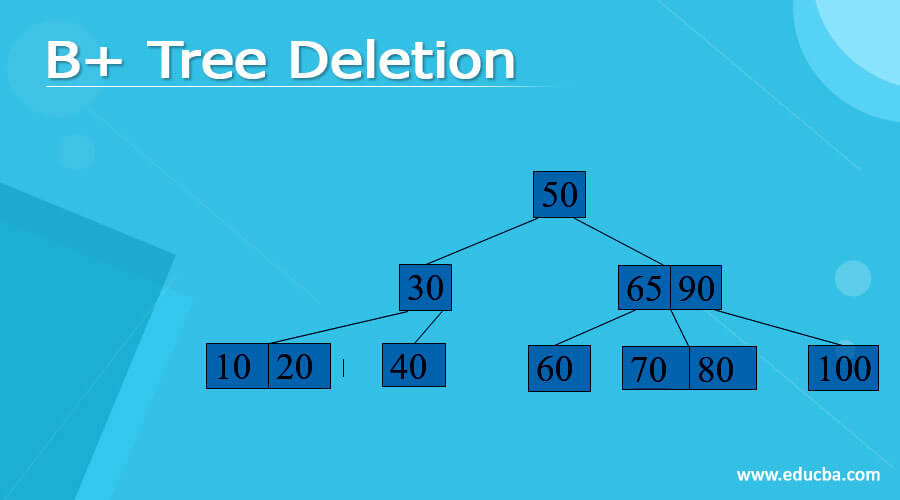## Definition of B+ Tree Deletion

B+ Tree is an extension of the B tree that allows more efficient insertion, deletion, and other operations than B tree. Deleting an element in the B+ tree includes three operations Searching, Deleting, and Balancing. First, we will search for the node that is to be deleted and performing a deletion operation on it then we will balance the tree as the final step.

### Algorithm of B+ Tree Deletion

Step 1: Take the input in a key-value and search for the leaf node containing the key value.

Step 2: If the key is found, remove that entry from the leaf

• If the leaf meets “Half Full criteria” then it is done
• otherwise, the leaf has some data entries.

Step 3: If the leaf’s right sibling can have an entry. then move the very smallest entry to that right sibling of the leaf.

• Otherwise, if the leaf’s left sibling can take an entry, then move the smallest node to that left sibling of the leaf.

• If it doesn’t meet the above two criteria, merge both leaf and a sibling.

Step 4: While merging, it recursively deletes the entry which is pointing to the leaf or sibling from the parent.

Step 5: Merging could make a change in the height of the tree.

### Explanation with an Example

Let us consider a B+ tree as shown below.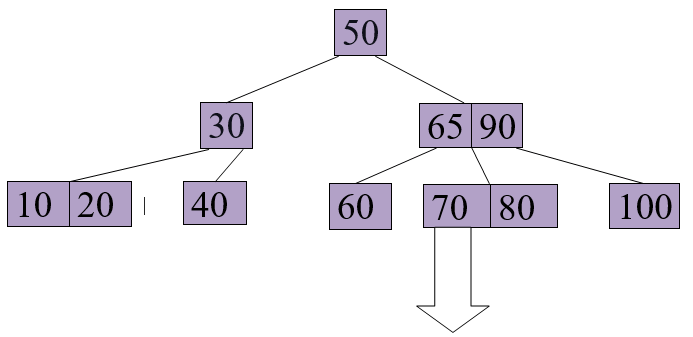Delete 65 from the above B+ tree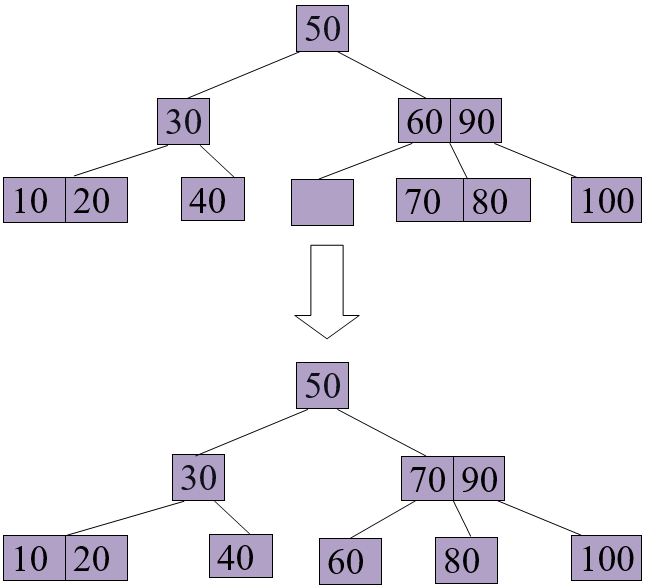Delete 70 from the above B+ tree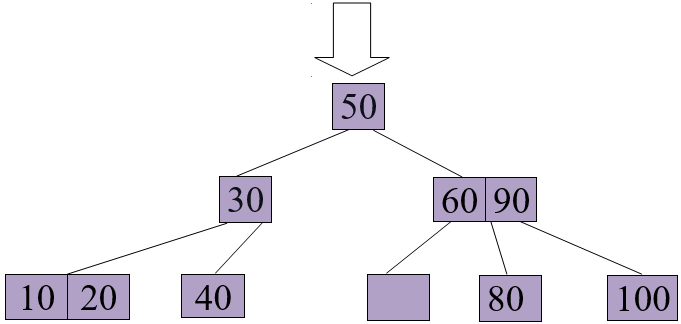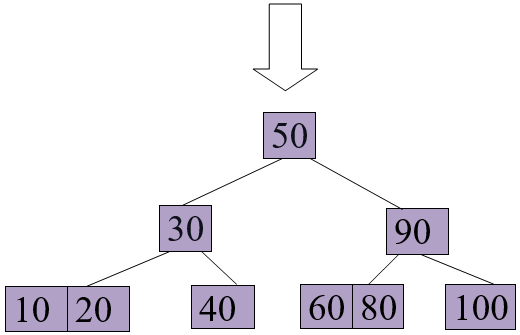Source Code

```import math # Node creation class Node: def __init__(self, order): self.order = order self.values = [] self.keys = [] self.nextKey = None self.parent = None self.check_leaf = False # Insert at the leaf def insert_at_leaf(self, leaf, value, key): if (self.values): temp1 = self.values for i in range(len(temp1)): if (value == temp1[i]): self.keys[i].append(key) break elif (value < temp1[i]): self.values = self.values[:i] + [value] + self.values[i:] self.keys = self.keys[:i] + [[key]] + self.keys[i:] break elif (i + 1 == len(temp1)): self.values.append(value) self.keys.append([key]) break else: self.values = [value] self.keys = [[key]] # B plus tree class BplusTree: def __init__(self, order): self.root = Node(order) self.root.check_leaf = True # Insert operation def insert(self, value, key): value = str(value) old_node = self.search(value) old_node.insert_at_leaf(old_node, value, key) if (len(old_node.values) == old_node.order): node1 = Node(old_node.order) node1.check_leaf = True node1.parent = old_node.parent mid = int(math.ceil(old_node.order / 2)) - 1 node1.values = old_node.values[mid + 1:] node1.keys = old_node.keys[mid + 1:] node1.nextKey = old_node.nextKey old_node.values = old_node.values[:mid + 1] old_node.keys = old_node.keys[:mid + 1] old_node.nextKey = node1 self.insert_in_parent(old_node, node1.values, node1) # Search operation for different operations def search(self, value): current_node = self.root while(current_node.check_leaf == False): temp2 = current_node.values for i in range(len(temp2)): if (value == temp2[i]): current_node = current_node.keys[i + 1] break elif (value < temp2[i]): current_node = current_node.keys[i] break elif (i + 1 == len(current_node.values)): current_node = current_node.keys[i + 1] break return current_node # Find the node def find(self, value, key): l = self.search(value) for i, item in enumerate(l.values): if item == value: if key in l.keys[i]: return True else: return False return False # Inserting at the parent def insert_in_parent(self, n, value, ndash): if (self.root == n): rootNode = Node(n.order) rootNode.values = [value] rootNode.keys = [n, ndash] self.root = rootNode n.parent = rootNode ndash.parent = rootNode return parentNode = n.parent temp3 = parentNode.keys for i in range(len(temp3)): if (temp3[i] == n): parentNode.values = parentNode.values[:i] + [value] + parentNode.values[i:] parentNode.keys = parentNode.keys[:i + 1] + [ndash] + parentNode.keys[i + 1:] if (len(parentNode.keys) > parentNode.order): parentdash = Node(parentNode.order) parentdash.parent = parentNode.parent mid = int(math.ceil(parentNode.order / 2)) - 1 parentdash.values = parentNode.values[mid + 1:] parentdash.keys = parentNode.keys[mid + 1:] value_ = parentNode.values[mid] if (mid == 0): parentNode.values = parentNode.values[:mid + 1] else: parentNode.values = parentNode.values[:mid] parentNode.keys = parentNode.keys[:mid + 1] for j in parentNode.keys: j.parent = parentNode for j in parentdash.keys: j.parent = parentdash self.insert_in_parent(parentNode, value_, parentdash) # Delete a node def delete(self, value, key): node_ = self.search(value) temp = 0 for i, item in enumerate(node_.values): if item == value: temp = 1 if key in node_.keys[i]: if len(node_.keys[i]) > 1: node_.keys[i].pop(node_.keys[i].index(key)) elif node_ == self.root: node_.values.pop(i) node_.keys.pop(i) else: node_.keys[i].pop(node_.keys[i].index(key)) del node_.keys[i] node_.values.pop(node_.values.index(value)) self.deleteEntry(node_, value, key) else: print("Value not in Key") return if temp == 0: print("Value not in Tree") return # Delete an entry def deleteEntry(self, node_, value, key): if not node_.check_leaf: for i, item in enumerate(node_.keys): if item == key: node_.keys.pop(i) break for i, item in enumerate(node_.values): if item == value: node_.values.pop(i) break if self.root == node_ and len(node_.keys) == 1: self.root = node_.keys node_.keys.parent = None del node_ return elif (len(node_.keys) < int(math.ceil(node_.order / 2)) and node_.check_leaf == False) or (len(node_.values) < int(math.ceil((node_.order - 1) / 2)) and node_.check_leaf == True): is_predecessor = 0 parentNode = node_.parent PrevNode = -1 NextNode = -1 PrevK = -1 PostK = -1 for i, item in enumerate(parentNode.keys): if item == node_: if i > 0: PrevNode = parentNode.keys[i - 1] PrevK = parentNode.values[i - 1] if i < len(parentNode.keys) - 1: NextNode = parentNode.keys[i + 1] PostK = parentNode.values[i] if PrevNode == -1: ndash = NextNode value_ = PostK elif NextNode == -1: is_predecessor = 1 ndash = PrevNode value_ = PrevK else: if len(node_.values) + len(NextNode.values) < node_.order: ndash = NextNode value_ = PostK else: is_predecessor = 1 ndash = PrevNode value_ = PrevK if len(node_.values) + len(ndash.values) < node_.order: if is_predecessor == 0: node_, ndash = ndash, node_ ndash.keys += node_.keys if not node_.check_leaf: ndash.values.append(value_) else: ndash.nextKey = node_.nextKey ndash.values += node_.values if not ndash.check_leaf: for j in ndash.keys: j.parent = ndash self.deleteEntry(node_.parent, value_, node_) del node_ else: if is_predecessor == 1: if not node_.check_leaf: ndashpm = ndash.keys.pop(-1) ndashkm_1 = ndash.values.pop(-1) node_.keys = [ndashpm] + node_.keys node_.values = [value_] + node_.values parentNode = node_.parent for i, item in enumerate(parentNode.values): if item == value_: p.values[i] = ndashkm_1 break else: ndashpm = ndash.keys.pop(-1) ndashkm = ndash.values.pop(-1) node_.keys = [ndashpm] + node_.keys node_.values = [ndashkm] + node_.values parentNode = node_.parent for i, item in enumerate(p.values): if item == value_: parentNode.values[i] = ndashkm break else: if not node_.check_leaf: ndashp0 = ndash.keys.pop(0) ndashk0 = ndash.values.pop(0) node_.keys = node_.keys + [ndashp0] node_.values = node_.values + [value_] parentNode = node_.parent for i, item in enumerate(parentNode.values): if item == value_: parentNode.values[i] = ndashk0 break else: ndashp0 = ndash.keys.pop(0) ndashk0 = ndash.values.pop(0) node_.keys = node_.keys + [ndashp0] node_.values = node_.values + [ndashk0] parentNode = node_.parent for i, item in enumerate(parentNode.values): if item == value_: parentNode.values[i] = ndash.values break if not ndash.check_leaf: for j in ndash.keys: j.parent = ndash if not node_.check_leaf: for j in node_.keys: j.parent = node_ if not parentNode.check_leaf: for j in parentNode.keys: j.parent = parentNode # Print the tree def printTree(tree): lst = [tree.root] level =  leaf = None flag = 0 lev_leaf = 0 node1 = Node(str(level) + str(tree.root.values)) while (len(lst) != 0): x = lst.pop(0) lev = level.pop(0) if (x.check_leaf == False): for i, item in enumerate(x.keys): print(item.values) else: for i, item in enumerate(x.keys): print(item.values) if (flag == 0): lev_leaf = lev leaf = x flag = 1 record_len = 3 bplustree = BplusTree(record_len) bplustree.insert('5', '33') bplustree.insert('15', '21') bplustree.insert('25', '31') bplustree.insert('35', '41') bplustree.insert('45', '10') printTree(bplustree) print() if(bplustree.find('5', '33')): print("Found") else: print("Not found") print() if(bplustree.find('5', '34')): print("Found") else: print("Not found")```

Output: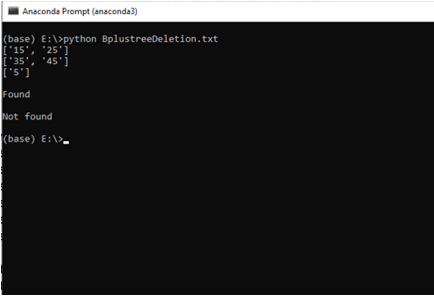### Time Complexity of B+ tree

• Best Case Time Complexity

The Best case time complexity of the deletion operation of a B+ tree is the same as the searching operation in a B+ tree. So, The best case time complexity of B+ tree deletion is Θ(logn)

• Average Case Time Complexity

The average case time complexity of B+ tree is Θ(logn). The deletion algorithm of B+ tree also takes the same time as performing search operation. So the time complexity of both search and Deletion will be equal.

• Worst Case Time Complexity

The worst-case time complexity of B+ tree is Θ(logn).

• Space Complexity of B+ tree

The average case space complexity and worst-case space complexity are equal in B+ tree.

The average case space complexity is Θ(n)

The best-case space complexity is Θ(n)

### Application of B+ tree

• In the B+ tree we store all the data in leaf nodes which makes the tree shorter and have more branching in these trees which reduces disk I/O. So we can implement these in Secondary storage devices.
• By using the B+ tree, one can retrieve partial retrieval or range retrieval. Traversing through the B+ tree makes this easier

• By using disc accesses, any records can be searched and accessed very easily.
• When compared to other trees, the height of B+ tree remains short and balanced.
• The data in B+ tree can be accessed sequentially or directly.
• As the data is stored in leaf nodes, it can be accessed fastly.

### Conclusion

• B+ Tree is an extension of the B tree that allows the more efficient insertion, deletion, and other operations than Btree
• Deleting an element in the B+ tree includes three operations Searching, Deleting, and Balancing
• If the height of the B+ tree gets shrunk it gets very complicated to continue further processes.

### Recommended Articles

This is a guide to B+ Tree Deletion. Here we discuss the definition, Algorithm of B+ Tree Deletion, explanation with example with code implementation and advantages. You may also have a look at the following articles to learn more –

The post B+ Tree Deletion appeared first on EDUCBA.